# Abstract

This paper presents an inversion methodology through weighted least squares to obtain the electrical parameters for the soil of a typical mid-western region in Brazil using the model based in the formalism of the parabolic equations to calculate the electric field intensity received. To validate this methodology, the results of the radio signal measurement campaign conducted in six radial routes leaving the city of Brasilia, Federal District, where the transmitter was located, were used. The measurements were compared to computer simulations and, thus, the optimal values for the electric conductivity and relative permittivity for the soil of the region could be estimated. Finally, a quantitative analysis of these parameters was performed with the values found in the literature, which demonstrated the effectiveness of the proposed methodology.

Index Terms
conductiv; electric field; inversion; parabolic equations; permittivity

# introduction

Since 2005, the Brazilian government, through the National Telecommunications Agency, ANATEL, has been authorizing digital signal transmission tests aimed at the implementation of the future Brazilian model for digital radio broadcast for frequencies below 30 MHz, in compliance with the recommendations of the Nr. 22 NTC Technical Standard. The standards of Digital Radio Mondiale (DRM) and High Definition (HD) were chosen for the tests because of the compatibility between their frequency bands and the current bands designed to radio broadcast in Brazil, thus allowing for the coexistence of both analog and digital systems.

Regardless of the standard that will be adopted, studying the behavior of the signal generated by the stations that will use the new systems for digital radio broadcasting in Brazil is required as well as the knowledge of the electrical parameters of the soil, especially in the Medium Wave band, where the effects of terrain strongly influence the determination of the electric field received and, therefore, the determination of the coverage area of these stations.

Thus, it is paramount to use appropriate values for the electrical parameters of the soil in the calculation of the electric field intensity during the planning of these systems, mainly because digital systems are much more sensitive, where small changes in the value of the electric field may cause total signal loss in the receiver.

This paper presents a method of inversion through weighted least squares to obtain the electrical parameters of the soil in a typical region in mid-western Brazil, which is characterized by highways or streets that cut through rural areas, where for this region, the World Atlas of Ground Conductivities of recommendation ITU-R P.832-2 1Rec. ITU-R P.832-2: ‘World atlas of ground conductivities’, 1999 recommends a value of 1 mS / m for the soil electrical conductivity.

The formulation of the inverse problem using the weighted least squares was considered because it is the form that more quickly leads to the convergence of results in the majority of the optimization techniques currently in use 2Habashy, T. M: ‘A General Framework for Constraint Minimization for the Inversion of Electromagnetic Measurements’ appeared as a chapter in the book series Progress in Electromagnetic Research, PIER 46, pp. 265-312, 2004.-3Habashy, T. M: ‘An inversion methodology using a weighted least- squares constrained minimization approach’. Research note, Schlumberger-Doll Research, 2003..

To analyze the methodology proposed herein, the results obtained in the campaign for electric field intensity measurement conducted over six radial routes leaving the city of Brasilia, Federal District, toward a rural region in mid-western Brazil 4Lima, F.F., Soares, A.J.M., Junior. A. and Silva, L.M. da: ‘Field Intensity Measure in Medium Waves for Broadcasting Digitalization Planning in Brazil’. IEEE AP-S/URSI/AMEREM 2006 Symposium. were used; also, the electric field was calculated through the method based on the formalism of the parabolic equations 5Levy, M.: ‘Parabolic Equation Methods for Electromagnetic Wave Propagation’, London: Institution of Electrical Engineers (United Kingdom, 2000, 1st edn.), pp. 1-236., whereas this method was used because it shows values very close to those obtained in measurements when compared to some of the classic models used in determining loss of propagation.

The measurement system consisted in a stationary laboratory transmitting a carrier at 980 kHz and two mobile units that were mounted to measure and store the instantaneous values of received signal strength. The results of measurements were compared with computational simulations through the method of inversion proposed.

# methodology

In the study of the behavior of a system through a mathematical model, it is essential to have knowledge of the various parameters involved, such as the information at the system input and boundary conditions. However, if some of these parameters are unknown, it is necessary to first consider the inverse problem, where the information about the response of the system will be available a priori. After this step, solving the direct problem is commenced.

By conducting measurement campaigns, the unknown parameters of the system can be found using the solution of the inverse problem, where determining a vector of unknown parameters that will minimize the difference between the measured values and the values obtained from the simulated response by a model that describes the system is attempted. This difference is known as the cost function, where minimization thereof will represent the optimization of said parameters.

Amongst the techniques used in the literature for the minimization of the cost function, this paper will consider Newton’s method.

A. Cost Function

For this paper, the cost function based on weighted least squares that have the following representation  was selected: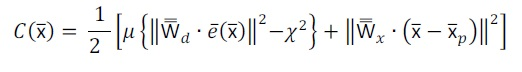(1)

where x̅ represents the vector of unknown parameters of the model, μ is a regularization factor, also known as Lagrange multiplier and e̅x̅ represents the vector of residuals. The term W̿d is the inverse of the data covariance matrix describing the variance represented by the weight of each measurement mj and estimated correlation existing between the errors. The parameter χ provides an a priori estimate of the noise present in the data. The term W̿x is the inverse of the covariance matrix of the model, given as a priori information and represents the importance of each element in the vector x̅.

The residual vector e̅x̅ is defined as a vector containing Melements representing the j-th error between the j-th measurement mj and the corresponding j-th response simulated by the model Sj. The normalized representation thereof is given by: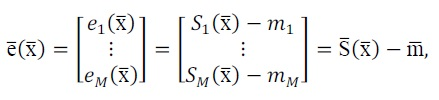(2)

B. Newton’s Method

The Newton's method is a quadratic model of the cost function and its representation through the first three terms of the Taylor series expansion around the k-th iteration x̅k is given by :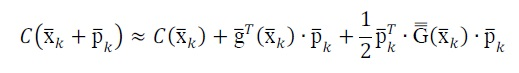(3)

where the step in x̅k toward the minimum of the cost function Cx̅ is: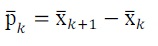(4)

and g̅x̅=∇Cx̅ is the gradient vector of the cost function defined as: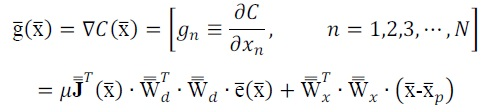(5)

Where J̿x̅ is the Jacobian matrix of order MXN given by: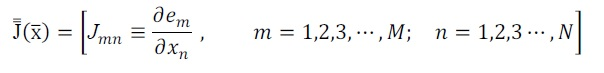(6)

and G̿x̅=∇∇Cx̅ is the Hessian of the cost functionCx̅ represented by:(7)

In the term Q̿x̅, the second order information of the cost function Cx̅ is represented.

The minimum of the cost function is achieved when the step p̅k is the minimum of the function below:(8)

Making the gradient ∅ to be null in p̅0: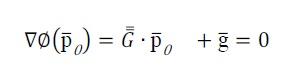(9)

By solving (9), a stationary point of the cost function is found, i.e., a point of minimum, maximum or saddle point in p̅k=p̅0. Thus, this stationary point is the solution to the following set of linear equations: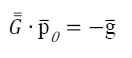(10)

In the implementation of the Newton’s method conducted herein, G̿x̅k was considered a nonsingular matrix and positive definite, which results in a unique solution to (10) given by:(11)

In this case, there is only one stationary point p̅0 in a descent direction, i.e., toward the minimum of the cost function, since:(12)

Substituting p̅0 in (3), the following is obtained: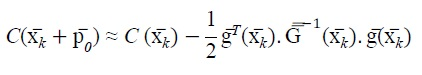(13)

The equation (13) represents the cost function at its minimum value, where x̅k is the optimal vector of unknown parameters of the model and p̅0 is known as Newton's search direction.

# parabolic equation model

To calculate the simulated response, the model based on the formalism of parabolic equations  was used herein. This model was chosen because it obtained values of electric field intensity much closer to the values obtained in the measurement campaign in the region 6Teixeira, A. S., Oliveira, Nascimento, R. A. de, Souza, J. F. de, Magno, F. N.B. and Cavalcante, G. P. ‘Electric Field Prediction for Medium Waves Radio Broadcasting Using Parabolic Equations’. In: International Microwave and Optoelectronics Conference, Natal. Anais IMOC 2011.. Moreover, this model uses lower computational effort 7Souza, J. F. de, F. N.B., Valente. Z. A., Costa, J. C. and Cavalcante, G. P. S. ‘Mobile Radio Propagation along Mixed Paths in Forest Environment using Parabolic Equation’, Microwave and Optical Technology Letters, Vol. 51 (4), April 2009, pp. 1133-1136.-8Oliveira, R. A. N. de, Souza, J. F. de, Magno, F. N. B., Cozzolino, K. and Cavalcante, G. P. S. ‘Propagation Loss Path Prediction Using Parabolic Equations for narrow and wide angles’, 7th European Conference on Antennas and Propagation (EUCAP) 2013, pp.944-948.[7-8].

Initially, an environment made up of small streets with buildings and vegetation was considered. Then, the method of parabolic equations was applied for calculating the electric field considering the electrical parameters involved in this simulated environment.

To the solution of the parabolic equation, the finite difference scheme of Crank-Nicolson was used for small angles (up to 20 degrees).

The parabolic equation for small angles is given by:(14)

Where k is the wave number and n is the refractive index given by the following expression 9Wangsness, R. K.: ‘Electromagnetic Fields’, United States of America: John Wiley & Sons, 1979, pp. 34 – appendices.:(15)

where εr is the relative permittivity, is the conductivity (S / m), f is the frequency (Hz), ε0 is the permittivity of free space (F / m).

Making the discretization of 14 in direction x and z through the finite difference scheme of Crank-Nicolson, where �

xm-1 to xm, the following is obtained 1010 Premat, E.: ‘Prise en Compte d´effets Météorologiques dans une Méthode d´Eléments finis de Frontière’, thèse de docteur, France, pp. 259-261, 2000.:(16)

To calculate the propagation loss through the parabolic equations method, the equation below was used:(17)

Where u0 is the electric field at a reference distance (d0), u is the received electric field, f is the frequency in GHz, and GT and GR are the gains of the transmitting antennas and receiving in dB, respectively.

# description of environment and measurement system

In order to validate the methodology of inversion presented in this paper, the results of the measurement campaigns of electric field intensity carried out by the National Telecommunications Agency - ANATEL - and by Brazilian Communications Company - Radiobrás in six radial routes from the city of Brasilia, Federal District, towards the cities of Passo (Route 1), Goiânia (Route 2), Niquelândia (Route 3), Arinos (Route 4 ), Crystal (route 5) and Goiás (route 6), where the environment of these routes is characterized by a typical rural region of centre of Brazil, were used. Fig. 1 illustrates this environment, highlighting the six radial routes.

Fig.1: Environment of measurements

The transmission system consisted in a Medium Wave generator, which was mounted at the Radiobras headquarters in Brasilia, located in Block 1, Lot S / N, in the Plano Piloto. Table I shows the characteristics of the transmitter.

TABLE I – CHARACTERISTICS OF TRANSMITTER.

For the measurements performed on routes 1, 2 and 6, the mobile unit of ANATEL was used, assembled in France by Thales Communications. This unit has equipment for the measurement of technical parameters, such as modulation type, frequency, electric field intensity, spectrum analysis and occupancy rates. Fig. 2 illustrates a block diagram of this unit.

Fig.2:The equipment setup in the mobile unit of ANATEL 1111 Li, L. W., Yeo, T. S., Kooi, P.S., Leong, M. S. P. and Koh J. H.: ‘Analysis of Electromagnetic Wave Propagation in Forest Environment along Multiple Paths’, Progress in Electromagnetism Research, PIER 23, 1999, pp. 137-164.

Initially, an AM signal was captured by the monopole antenna RN4203 that sent it to the switch of antenna AEA196. Then, the signal was sent off to the REC108 receptor, which has two modules, the first of which translates the signal of radio frequency (RF) to intermediate frequency (IF), and the second one samples and demodulates the signal. After this step, the signal samples were sent to the central processing unit KPRII, which obtained the values of the electric field intensity for each sample of the signal at intermediate frequency.

For the collection and storage of the geographic coordinates for the samples of the received signal in IF, a Trimble GPS connected to the RS232 of KPRII was used.

Also, a handheld GPS connected to a Palm Top was used for registering the geographic coordinates of the places where there occurred abrupt changes in the intensity value of the measured electric field.

For the measurement campaign on routes 3, 4 and 5, a mobile unit of Radiobras was used, with the participation of members of the consortium DRM (Digital Radio Mondiale). Fig. 3 shows the measurement system installed on this mobile unit.

Fig.3:The equipment setup in the mobile unit of Radiobras 1212 Lima, F. F., Soares, A.J.M., Abdalla, H.A. and Silva, L. M.: ‘Methodology for the Determination of the Ground’s Electric Conductivity in rural Environments trough Measurements of the Surface Wave’, The First European Conference on Antennas and Propagation, Nice, France, 2006.

This mobile unit had a short monopole antenna, model HE010, which received an AM signal and delivered it to a PSU (Power Supply Unit). Then, the signal was send to the EB200 receiver for translating the RF band to the IF band and baseband. After this step, the signal followed to a 24-bit Creative Sound Blaster sound card, which was connected to a USB port on a Lap Top. This Lap Top contained the Dream DRM and TSR software that demodulated the signal and captured of the value of the electric field intensity.

For each sample obtained by TSR DRM software, the value of the electric field intensity received was stored. Also, information of the geographic coordinates of each sample measured using a GPS was stored.

# results

This section presents the results obtained from the use of the inversion methodology through weighted least squares in the estimation of the electrical parameters of the soil in a region in mid-western Brazil.

This region consists of a rural environment where radio signal measurements were conducted in six radial routes leaving the city of Brasilia, Federal District, where a transmitter was installed in a building of the Brazilian Communications Company located in the center of Brasilia, which emitted an amplitude-modulated signal by a collinear antenna positioned 94m high.

For the measurement campaign, two mobile units were used: one of ANATEL (routes 1, 2 and 6) and another of Radiobras (routes 3, 4 and 5).

The Visual FORTRAN 6.0 program was used both to obtain the values of electric field intensity and for the numerical simulation of the inversion method to estimate the electrical parameters of the soil. A vector x̅ of unknown parameters of the model was set, which consisted of two elements: soil electrical conductivity and relative permittivity of the soil. The value of the Lagrange multiplier used was 1 x 10-8.

TABLE II. ELECTRICAL PARAMETERS ESTIMATED TO SOIL

After application of the inversion method proposed herein, the values for soil electrical conductivity and relative soil permittivity were calculated and considered to be homogeneous over the six routes shown in Table II.

In Table II, the estimated values for the electrical conductivity of soil in the six routes are can be seen to be much higher than the value recommended by the ITU for the region in mid-western Brazil. However, they are very close to the results found by LIMA  who developed a methodology that estimated the soil electrical conductivity by comparing the measured values of the attenuation of the signal with the values found through the Earth Spherical model for surface wave.

For the relative permittivity of the soil, the values estimated by using the inversion methodology in the six routes considered were verified to be slightly higher than the value adopted in , indicating the existence of a medium-type soil.

TABLE III – ELECTRICAL CONDUCTIVITY OF SOIL AND RELATIVE ERRORS

In order to evaluate the performance of the proposed model, Table III shows the optimal values of soil conductivity estimated by using the inversion methodology and those obtained in reference ; also, the relative errors between the two methods for each one of the six routes are presented.

In Table IIITable III, it can be observed that the values of the soil electrical conductivity for routes 1, 2, 5 and 6 are very close to the values obtained in reference  producing small relative errors, which are slightly larger for routes 3 and 4.

These values for the conductivity of the soil are also very close to those found in reference 1313 Silva, A. T. da, ‘Predição dos Campos Elétricos na Faixa de Ondas Médias em Ambiente Rural Utilizando Equações Parabólicas’, Dissertação de Mestrado, PPGEE/ITEC/UFPA, Brasil, PP. 1-72, 2011., where the same routes studied here through a methodology based on Artificial Neural Networks were analyzed.

# conclusion

This paper presented a methodology of inversion through weighted least squares to obtain the electrical parameters of the soil in a region in mid-western Brazil. Also, a comparative analysis between the values of the soil electrical conductivity through this methodology and the values found in the literature for the region was carried out.

To validate this methodology, the results of the measurement campaigns of the intensity of the electric field in six radial routes leaving the city of Brasilia bound for the neighboring cities were used, in an environment characterized by a typical rural region of mid-western Brazil. The electric field through the formalism based on the parabolic equation model was also calculated, since this model showed good concordance with the values obtained from the measurement campaigns.

The electrical parameters of the soil were estimated for the six routes studied using the inversion methodology presented, whereby the values obtained for the electrical conductivity of the soil were found to be higher than those recommended by the ITU, thus indicating that the coverage areas of local radio stations would be much higher than those established by ANATEL.

These estimated values for the electrical conductivity in the six routes were also found to be very close to the values found in recent studies conducted in the rural region in mid-western Brazil [12-13], showing small relative errors in the routes 1, 2, 5 and 6. Similarly, the values for the relative permittivity of the soil in the six routes were close to those adopted in the region.

Thus, it can be concluded that, by using the inversion method presented herein, it will be possible to find suitable values for soil electrical conductivity and relative permittivity of the soil, thus making it much easier to determine the electric field received with greater precision.

For future papers, obtaining the electric parameters of the soil, considering it inhomogeneous in each route, is suggested, and studying the changes in the values of electrical parameters with distance is also proposed.

# acknowledgment

The authors would like to thank the National Institute of Science and Technology – Wireless Communication (INCT-CSF) for its support.

# references

• 1
Rec. ITU-R P.832-2: ‘World atlas of ground conductivities’, 1999
• 2
Habashy, T. M: ‘A General Framework for Constraint Minimization for the Inversion of Electromagnetic Measurements’ appeared as a chapter in the book series Progress in Electromagnetic Research, PIER 46, pp. 265-312, 2004.
• 3
Habashy, T. M: ‘An inversion methodology using a weighted least- squares constrained minimization approach’. Research note, Schlumberger-Doll Research, 2003.
• 4
Lima, F.F., Soares, A.J.M., Junior. A. and Silva, L.M. da: ‘Field Intensity Measure in Medium Waves for Broadcasting Digitalization Planning in Brazil’. IEEE AP-S/URSI/AMEREM 2006 Symposium.
• 5
Levy, M.: ‘Parabolic Equation Methods for Electromagnetic Wave Propagation’, London: Institution of Electrical Engineers (United Kingdom, 2000, 1st edn.), pp. 1-236.
• 6
Teixeira, A. S., Oliveira, Nascimento, R. A. de, Souza, J. F. de, Magno, F. N.B. and Cavalcante, G. P. ‘Electric Field Prediction for Medium Waves Radio Broadcasting Using Parabolic Equations’. In: International Microwave and Optoelectronics Conference, Natal. Anais IMOC 2011.
• 7
Souza, J. F. de, F. N.B., Valente. Z. A., Costa, J. C. and Cavalcante, G. P. S. ‘Mobile Radio Propagation along Mixed Paths in Forest Environment using Parabolic Equation’, Microwave and Optical Technology Letters, Vol. 51 (4), April 2009, pp. 1133-1136.
• 8
Oliveira, R. A. N. de, Souza, J. F. de, Magno, F. N. B., Cozzolino, K. and Cavalcante, G. P. S. ‘Propagation Loss Path Prediction Using Parabolic Equations for narrow and wide angles’, 7th European Conference on Antennas and Propagation (EUCAP) 2013, pp.944-948.
• 9
Wangsness, R. K.: ‘Electromagnetic Fields’, United States of America: John Wiley & Sons, 1979, pp. 34 – appendices.
• 10
Premat, E.: ‘Prise en Compte d´effets Météorologiques dans une Méthode d´Eléments finis de Frontière’, thèse de docteur, France, pp. 259-261, 2000.
• 11
Li, L. W., Yeo, T. S., Kooi, P.S., Leong, M. S. P. and Koh J. H.: ‘Analysis of Electromagnetic Wave Propagation in Forest Environment along Multiple Paths’, Progress in Electromagnetism Research, PIER 23, 1999, pp. 137-164.
• 12
Lima, F. F., Soares, A.J.M., Abdalla, H.A. and Silva, L. M.: ‘Methodology for the Determination of the Ground’s Electric Conductivity in rural Environments trough Measurements of the Surface Wave’, The First European Conference on Antennas and Propagation, Nice, France, 2006.
• 13
Silva, A. T. da, ‘Predição dos Campos Elétricos na Faixa de Ondas Médias em Ambiente Rural Utilizando Equações Parabólicas’, Dissertação de Mestrado, PPGEE/ITEC/UFPA, Brasil, PP. 1-72, 2011.

# Publication Dates

• Publication in this collection
June 2015